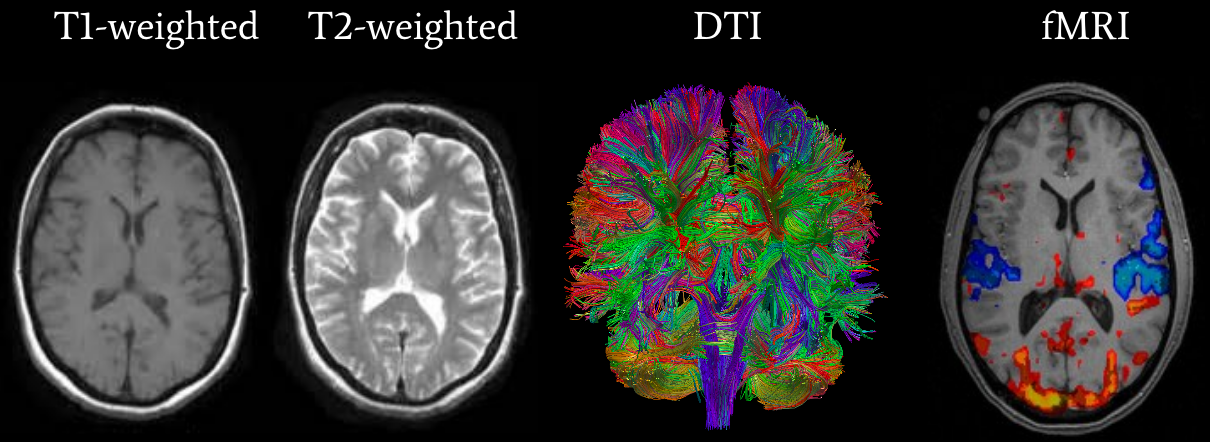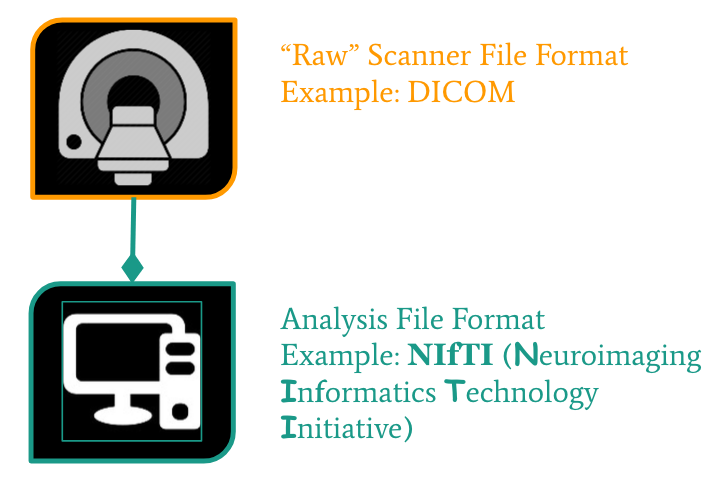This lesson is in the early stages of development (Alpha version)

# Neuroimaging Fundamentals & Nibabel

## Overview

Teaching: 20 min
Exercises: 10 min
Questions
• How are images loaded in Python?

Objectives
• Lean about imaging data structures.

## Why Python?

• free, open source
• one platform for data pre-processing, visualization and analysis
• reproducible code
• large number of user-developed packages (eg. nibabel, nilearn)
• easy interaction with state-of-the art neuroimaging software (eg. FSL, ANTS)

## Types of MR ScansFor this tutorial, we’ll be focusing on T1w and resting state fMRI scans.

## Neuroimaging File Formats

Format Name File Extension Origin
Analyze .img/.hdr Analyze Software, Mayo Clinic
DICOM none ACR/NEMA Consortium
NIfTI .nii or .img/.hdr Neuroimaging Informatics Technology Initiative
MINC .mnc Montreal Neurological Institute
NRRD .nrrdFrom the MRI scanner, images are initially collected in the DICOM format and can be converted to NIfTI using dcm2niix.

## Intro to NIfTI

NIfTI is one of the most ubiquitous file formats for storing neuroimaging data. We’ll cover a few details to get started working with them. If you’re interested in learning more about NIfTI images, we highly recommend this blog post about the NIfTI format.

NiBabel is a Python package for reading and writing neuroimaging data. To learn more about how NiBabel handles NIfTIs, check out the Working with NIfTI images page of the NiBabel documentation.

import nibabel as nib


First, use the load() function to create a NiBabel image object from a NIfTI file. We’ll load in a T1w image from the dataset we’ll be using for this tutorial.

t1_img = nib.load('../data/ds000030/sub-10788/anat/sub-10788_T1w.nii.gz')
type(t1_img)


There are three main components of a NIfTI image:

t1_hdr = t1_img.header


You can easily access specific metadata from the NiBabel image header object through dictionary keys.

t1_hdr.keys()


## Exercise #1

Extract the value of pixdim from t1_hdr

## Solution

t1_hdr['pixdim']


4

### 2. Data

The data is a multidimensional array representing the image data.

t1_data = t1_img.get_data()
t1_data


The data is stored in a numpy array.

type(t1_data)


We can check some basic properties of the array.

## Exercise #2

How many dimensions does t1_data have? What is the size of each dimension? What is the data type?

## Solution

t1_data.ndim
t1_data.shape
t1_data.dtype



4

The shape of the data always has at least 3 dimensions (X, Y, and Z) and sometimes a 4th, T (time).
This T1w image has 3 dimensions. The brain was scanned in 176 slices with a resolution of 256 x 256 voxels per slice.

The data type of an image controls the range of possible intensities. As the number of possible values increases, the amount of space the image takes up in memory also increases.

Data Type Range Number of Values
uint8 0, 255 256
uint16 -128, 127 256
uint 16 0, 2^16 2^16
int16 -2^15, 2^15 2^16
float16 ~-2^16, ~2^16 »2^16

### 3. Affine: tells the position of the image array data in a reference space

The affine array tells the position of the image array data in a reference space. It translates between data-space and world-space.

t1_affine = t1_img.affine
t1_affine


<div class=exercise> EXERCISE: Explore some of the other methods that can be called on the NIfTI image. </div>

## Working With Image Data

### Slicing

n-dimensional images are just stacks of numpy arrays. Each value in the array is assigned to an x, y or z coordinate.You’ll recall our example T1w image is a 3D image with dimensions $176 \times 256 \times 256$.

## Exercise #3

Select the central slice by indexing t1_data eg.(t1_data[x, y, z])

## Solution

central_slice = t1_data[t1_data.shape//2 - 1, :, :]
central_slice



4

Instead of indexing, we can also call slicer() central_slice = t1_img.slicer[87:88, :, :].get_data() central_slice

### Visualizing

Let’s visualize the central slice.

import matplotlib.pyplot as plt
%matplotlib inline

plt.imshow(central_slice, cmap='gray')


You’ll notice that the image is rotated. :( Don’t worry, we can fix this!

import numpy as np

rot_central_slice = np.rot90(central_slice, k=1)
plt.imshow(rot_central_slice, cmap='gray')


You’ll notice that so far, we’ve only seen a sagittal slice. Lets visualize the sagittal, axial and coronal slices.

# function to display a row of slices
def show_slices(slices):
""" Function to display row of image slices """
fig, axes = plt.subplots(1, len(slices))
for i, slice in enumerate(slices):
axes[i].imshow(slice.T, cmap="gray", origin="lower")
for ax in axes:
ax.axis('off')

slice_0 = t1_data[87, :, :]
slice_1 = t1_data[:, 127, :]
slice_2 = t1_data[:, :, 127]
show_slices([slice_0, slice_1, slice_2])
plt.suptitle("Center slice")


All this is fine but NiBabel makes it even easier to visualize all three planes. Call the orthoview() method on the NiBabel image object.

t1_img.orthoview()


### Reshaping

NumPy has a reshape() function for reshaping the data array. Let’s say we want to convert this 3D array into a a 2D array.

t1_data_2d = t1_data.reshape(np.prod(t1_data.shape[:-1]), t1_data.shape[-1])
t1_data_2d.shape


Next, we will see how to segment the brain from the black background.

plt.hist(t1_data.flatten(), bins = 50)

t1_mask = t1_data > 100

plt.imshow(t1_mask[87, :, :], cmap = 'gray')

test = np.where(t1_mask, t1_data, 0)
plt.imshow(test[87, :, :], cmap = 'gray')


### Writing NIfTI Images

Let’s save the mask we just created to a file.

img_mask = nib.Nifti1Image(test, t1_affine, t1_hdr)

img_mask.to_filename('../data/test_mask.nii.gz')


• blah